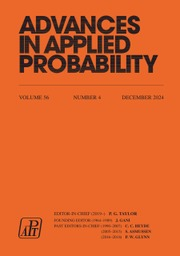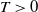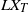Skip to main content Accessibility help
Home
Hostname: page-component-564cf476b6-dr4jh Total loading time: 0.283 Render date: 2021-06-20T05:32:44.270Z Has data issue: true Feature Flags: { "shouldUseShareProductTool": true, "shouldUseHypothesis": true, "isUnsiloEnabled": true, "metricsAbstractViews": false, "figures": true, "newCiteModal": false, "newCitedByModal": true, "newEcommerce": true }Advances in Applied Probability

# Simulation of elliptic and hypo-elliptic conditional diffusions

Published online by Cambridge University Press:  29 April 2020

Corresponding
E-mail address:

## Abstract

Suppose X is a multidimensional diffusion process. Assume that at time zero the state of X is fully observed, but at time$T>0$ only linear combinations of its components are observed. That is, one only observes the vector$L X_T$ for a given matrix L. In this paper we show how samples from the conditioned process can be generated. The main contribution of this paper is to prove that guided proposals, introduced in , can be used in a unified way for both uniformly elliptic and hypo-elliptic diffusions, even when L is not the identity matrix. This is illustrated by excellent performance in two challenging cases: a partially observed twice-integrated diffusion with multiple wells and the partially observed FitzHugh–Nagumo model.

## MSC classification

Type
Original Article
Information
Advances in Applied Probability , March 2020 , pp. 173 - 212
Copyright
© Applied Probability Trust 2020

## Access options

Get access to the full version of this content by using one of the access options below.

## References

Agarwal, R. P., Deng, S. andZhang, W. (2005). Generalization of a retarded Gronwall-like inequality and its applications. Appl. Math. Comput. 165, 599612.Google Scholar
Arnaudon, A., Holm, D. D. andSommer, S. (2019). A geometric framework for stochastic shape analysis. Found. Comput. Math. 19, 653701.CrossRefGoogle Scholar
Bayer, C. andSchoenmakers, J. (2014). Simulation of forward–reverse stochastic representations for conditional diffusions. Ann. Appl. Prob. 24 (5), 19942032.CrossRefGoogle Scholar
Beskos, A., Papaspiliopoulos, O., Roberts, G. O. andFearnhead, P. (2006). Exact and computationally efficient likelihood-based estimation for discretely observed diffusion processes. J. R. Statist. Soc. B Statist. Methodology 68, 333382.CrossRefGoogle Scholar
Beskos, A., Roberts, G., Stuart, A. andVoss, J. (2008). MCMC methods for diffusion bridges. Stoch. Dynamics 8, 319350.CrossRefGoogle Scholar
Bezanson, J., Karpinski, S., Shah, V. B. andEdelman, A. (2012). Julia: a fast dynamic language for technical computing. Available at arXiv:1209.5145.Google Scholar
Bladt, M., Finch, S. andSØrensen, M. (2016). Simulation of multivariate diffusion bridges. J. R. Statist. Soc. B. Statist. Methodology 78, 343369.CrossRefGoogle Scholar
Brockwell, P. (1994). On continuous-time threshold ARMA processes. J. Statist. Planning Infer. 39, 291303.CrossRefGoogle Scholar
Clairon, Q. andSamson, A. (2017). Optimal control for estimation in partially observed elliptic and hypoelliptic stochastic differential equations. Working paper, available at https://hal.archives-ouvertes.fr/hal-01621241/.Google Scholar
Clark, J. M. C. (1990). The simulation of pinned diffusions. In Proceedings of the 29th IEEE Conference on Decision and Control, 1990, pp. 14181420. IEEE.CrossRefGoogle Scholar
Comte, F., Prieur, C. andSamson, A. (2017). Adaptive estimation for stochastic damping Hamiltonian systems under partial observation. Stoch. Process. Appl. 127, 36893718.CrossRefGoogle Scholar
Cotter, S. L., Roberts, G. O., Stuart, A. M. andWhite, D. (2013). MCMC methods for functions: modifying old algorithms to make them faster. Statist. Sci. 28, 424446.CrossRefGoogle Scholar
Delyon, B. andHu, Y. (2006). Simulation of conditioned diffusion and application to parameter estimation. Stoch. Process. Appl. 116, 16601675.CrossRefGoogle Scholar
Ditlevsen, S. andSamson, A. (2019). Hypoelliptic diffusions: discretization, filtering and inference from complete and partial observations. J. R. Statist. Soc. B 81 (2), 361384.CrossRefGoogle Scholar
Durham, G. B. andGallant, A. R. (2002). Numerical techniques for maximum likelihood estimation of continuous-time diffusion processes. J. Bus. Econom. Statist. 20, 297338.CrossRefGoogle Scholar
Golightly, A. andWilkinson, D. J. (2006). Bayesian sequential inference for nonlinear multivariate diffusions. Statist. Comput. 16, 323338.CrossRefGoogle Scholar
Hairer, M., Stuart, A. M. andVoss, J. (2009). Sampling conditioned diffusions. In Trends in Stochastic Analysis (London Math. Soc. Lecture Note Series 353), pp. 159186. Cambridge University Press.CrossRefGoogle Scholar
Hairer, M., Stuart, A. M. andVoss, J. (2011). Sampling conditioned hypoelliptic diffusions. Ann. Appl. Prob. 21, 669698.CrossRefGoogle Scholar
Hermes, H. and LaSalle, J. P. (1969). Functional Analysis and Time Optimal Control. (Mathematics in Science and Engineering 56). Academic Press, New York and London.Google Scholar
Karatzas, I. andShreve, S. E. (1991). Brownian Motion and Stochastic Calculus, 2nd edn (Graduate Texts in Mathematics 113). Springer, New York.Google Scholar
Lin, M., Chen, R. andMykland, P. (2010). On generating Monte Carlo samples of continuous diffusion bridges. J. Amer. Statist. Assoc. 105, 820838.CrossRefGoogle Scholar
LindstrÖm, E. (2012). A regularized bridge sampler for sparsely sampled diffusions. Statist. Comput. 22, 615623.CrossRefGoogle Scholar
Liptser, R. S. andShiryaev, A. N. (2001). Statistics of Random Processes I: General Theory, expanded edn (Applications of Mathematics: Stochastic Modelling and Applied Probability 5). Springer, Berlin.Google Scholar
Lu, F., Lin, K. andChorin, A. (2016). Comparison of continuous and discrete-time data-based modeling for hypoelliptic systems. Commun. Appl. Math. Comput. Sci. 11, 187216.CrossRefGoogle Scholar
Mao, X. (1992). Almost sure polynomial stability for a class of stochastic differential equations. Quart. J. Math. Oxford Ser. (2) 43, 339348.CrossRefGoogle Scholar
Mao, X. (1997). Stochastic Differential Equations and their Applications (Horwood Publishing Series in Mathematics & Applications). Horwood Publishing, Chichester.Google Scholar
Marchand, J.-L. (2012). Conditionnement de processus markoviens. Doctoral thesis, IRMAR, Université de Rennes 1.Google Scholar
Melnykova, A. (2018). Parametric inference for multidimensional hypoelliptic diffusion with full observations. Available at arXiv:1802.02943.Google Scholar
Mider, M. andSchauer, M. (2019). BridgeSDEInference 0.1.1. doi:10.5281/zenodo.3446185.CrossRefGoogle Scholar
Papaspiliopoulos, O., Roberts, G. O. andStramer, O. (2013). Data augmentation for diffusions. J. Comput. Graph. Statist. 22, 665688.CrossRefGoogle Scholar
Pokern, Y., Stuart, A. M. andWiberg, P. (2009). Parameter estimation for partially observed hypoelliptic diffusions. J. R. Statist. Soc. B Statist. Methodology 71, 4973.CrossRefGoogle Scholar
Revuz, D. andYor, M. (1991). Continuous Martingales and Brownian Motion (Grundlehren der Mathematischen Wissenschaften 293). Springer, Berlin.Google Scholar
Samson, A. andThieullen, M. (2012). A contrast estimator for completely or partially observed hypoelliptic diffusion. Stoch. Process. Appl. 122, 25212552.CrossRefGoogle Scholar
Schauer, M.et al. (2018). Bridge 0.9.0. doi:10.5281/zenodo.1406163.CrossRefGoogle Scholar
Schauer, M., van der Meulen, F. andvan Zanten, H. (2017). Guided proposals for simulating multi-dimensional diffusion bridges. Bernoulli 23, 29172950.CrossRefGoogle Scholar
SØrensen, M. (2012). Estimating functions for diffusion-type processes. In Statistical Methods for Stochastic Differential Equations (Monogr. Statist. Appl. Probab. 124), pp. 1107. CRC Press, Boca Raton.CrossRefGoogle Scholar
Stramer, O. andRoberts, G. O. (2007). On Bayesian analysis of nonlinear continuous-time autoregression models. J. Time Ser. Anal. 28, 744762.CrossRefGoogle Scholar
van der Meulen, F. andSchauer, M. (2017). Bayesian estimation of discretely observed multi-dimensional diffusion processes using guided proposals. Electron. J. Statist. 11, 23582396.CrossRefGoogle Scholar
van der Meulen, F. andSchauer, M. Continuous-discrete smoothing of diffusions. Available at arXiv:1712.03807 2017.Google Scholar
van der Meulen, F. andSchauer, M. (2018). Bayesian estimation of incompletely observed diffusions. Stochastics 90, 641662.CrossRefGoogle Scholar
van der Meulen, F. andSchauer, M. (2019). Code examples hypoelliptic diffusions 0.1.0. doi:10.5281/zenodo.3457570, https://github.com/mschauer/code-examples-hypoelliptic-diffusions.Google Scholar
Whitaker, G. A., Golightly, A., Boys, R. J. andSherlock, C. (2017). Improved bridge constructs for stochastic differential equations. Statist. Comput. 27, 885900.CrossRefGoogle Scholar
Williams, D. (1981). To begin at the beginning: … In Stochastic Integrals (Lecture Notes Math. 851), pp. 155. Springer, Berlin and New YorkGoogle Scholar
1
Cited by

# Send article to Kindle

To send this article to your Kindle, first ensure no-reply@cambridge.org is added to your Approved Personal Document E-mail List under your Personal Document Settings on the Manage Your Content and Devices page of your Amazon account. Then enter the ‘name’ part of your Kindle email address below. Find out more about sending to your Kindle. Find out more about sending to your Kindle.

Note you can select to send to either the @free.kindle.com or @kindle.com variations. ‘@free.kindle.com’ emails are free but can only be sent to your device when it is connected to wi-fi. ‘@kindle.com’ emails can be delivered even when you are not connected to wi-fi, but note that service fees apply.

Find out more about the Kindle Personal Document Service.

Simulation of elliptic and hypo-elliptic conditional diffusions
Available formats
×

# Send article to Dropbox

To send this article to your Dropbox account, please select one or more formats and confirm that you agree to abide by our usage policies. If this is the first time you use this feature, you will be asked to authorise Cambridge Core to connect with your <service> account. Find out more about sending content to Dropbox.

Simulation of elliptic and hypo-elliptic conditional diffusions
Available formats
×

# Send article to Google Drive

To send this article to your Google Drive account, please select one or more formats and confirm that you agree to abide by our usage policies. If this is the first time you use this feature, you will be asked to authorise Cambridge Core to connect with your <service> account. Find out more about sending content to Google Drive.

Simulation of elliptic and hypo-elliptic conditional diffusions
Available formats
×
×

#### Reply to:Submit a response

Please enter your response.

#### Your details

Please enter a valid email address.

#### Conflicting interests

Do you have any conflicting interests? *